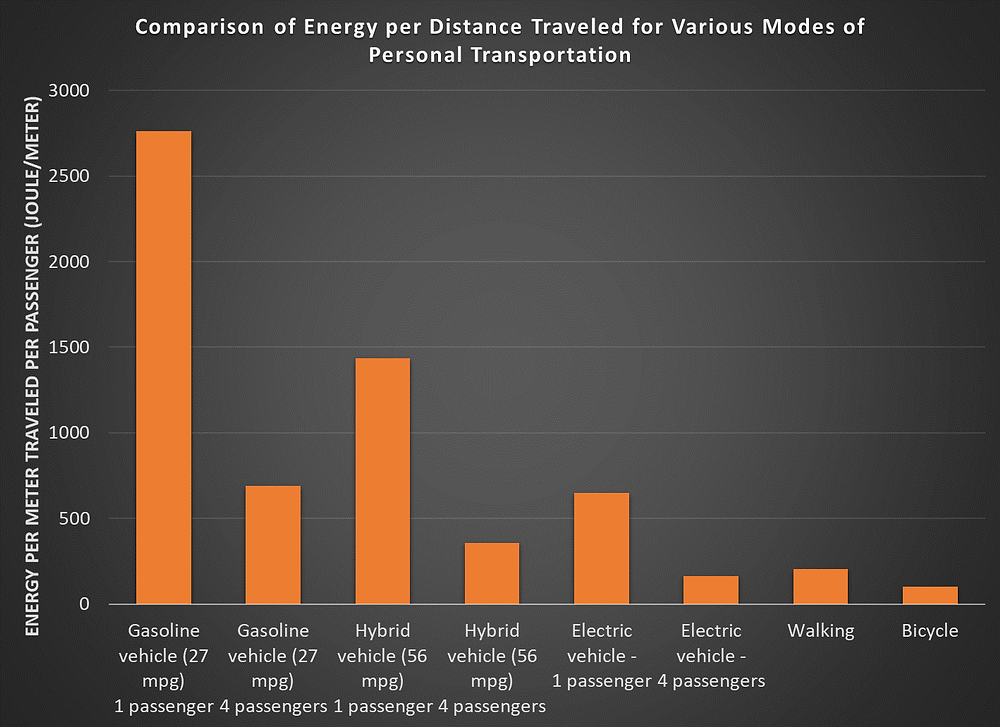Search
•Jeff LeBlanc

# Comparing efficiencies for different modes of transportation

The commonly used units for energy efficiencies for different modes of transportation make their comparisons less straightforward. For instance, vehicles with internal combustion engines (ICE) typically use miles per gallon (mpg) while electric vehicles (EVs) use watt-hours per mile. Meanwhile, human-propelled modes of transportation, like walking and riding a bicycle, depending on the food you eat, your weight, and your speed. Therefore, it can be quite illustrative to calculate the energy per distance for each mode of personal transportation, like Joules per meter.

For gasoline power vehicles, you can convert from mpg to Joules per meter starting from the energy content of 1 gallon of gasoline which is approximately 120 million Joules. Divide that number by the car’s average mpg then divide that number by 1609 meters per mile. The calculation for a car that gets 27 mpg is shown below;

120,000,000 Joules/gallon ÷ 27 miles/gallon ÷ 1,609 mile/meters = 2,764 Joules/meter

For EVs, you would need to start from the average Watt-hours per mile for the vehicles. A watt-hour is equivalent to the energy of 1 Joule used for 3,600 seconds. So, converting 290 Watt-hours per mile for an EV (like the Tesla Model S)to Joules per meter would look like this;

290 (Watt-hour)/mile × 3600 seconds/hour ÷ 1,609 mile/meters = 649 Joules/meter

Body mass can be used to predict energy expenditure with reasonable accuracy at walking speeds of 2 to 4 mph.  If your body mass is 141 pounds and you walk at a speed of 3.5 mph then you will burn approximately 4.6 calories per minute (4.6 x 60 minutes = 276 Calories). Converting calories to Joules and dividing by the distance traveled in meters, you’ll get 205 J/m.

As it turns out, you can travel about twice as fast on a bike by expending the same amount of energy as walking. So, the energy per meter of transportation by bicycle is about 100 J/m, roughly half that of walking.

The figure below summarizes these results.So, here is an “apples to apples” comparison of the energy per distance for various modes of personal transportation. At the extremes of the plot, you have gasoline-powered vehicles with only one passenger and bicycling. You should notice how car-pooling dramatically improves the energy efficiency of transportation. Due to four people traveling as opposed to one the energy per meter is cut-down by a factor of four. Furthermore, you may notice hybrid vehicles are about twice as efficient as ICE vehicles and EVs are MORE THAN twice as efficient as hybrids and MORE THAN four times as efficient as ICE vehicles! Once you start car-pooling in an EV then your energy efficiency is comparable to that of walking or riding a bike. If riding a bike is your main mode of transportation then good-job, you are one efficient traveler!

However, you still might ask, are electric vehicles still the more efficient automobile when you take the electricity generation into consideration?

The answer is yes, and I will show you why. In the table below, I have provided some efficiencies that we will need in the calculation. So, between your energy source and your electric car moving, there are a few components; the charger, inverter, battery, and (finally) motor drive train. The efficiency of each component is pretty darn good (≥90%) but, you need to multiply these all together to get the overall efficiency of making your EV go. That overall efficiency comes out to be 75.5% (or 76% due to sig. figs. 😊)

Now for the electricity generation component; electricity transmission is about 93% efficient (on average). The efficiency of electricity generation from coal or nuclear is 35% and that of electricity from natural gas combine cycle is 60%. Let’s combine each of these efficiencies with the efficiency of the EV;

For coal/nuclear, the combined efficiency from generation to traveling in your EV is;

75.5% ×93.0% ×35.0%=24.6%

For natural gas combined cycle, the combined efficiency from generation to traveling in your EV is;

75.5% ×93.0% ×60.0%=42.2%

(Notice here how much the method of generation affects your efficiency, that’s a swing of 17.6% between these two cases!)

Now, take the 649 Joules/meter for the EV and divide that by each efficiency;

(649 Joules/Meter)/(24.6%)=2,640 Joules/meter < 2,764 Joules/meter for ICE @ 27 mpg

And

(649 Joules/Meter)/(42.2%)=1,540 Joules/meter2,764 Joules/meter for ICE @ 27 mpg

So, there you have it. In both cases, the EV is more efficient than the ICE vehicle getting 27 mpg. Granted, given an area where electricity generation is from coal or nuclear, the efficiency of an ICE or hybrid vehicle with 29 mpg or better would best that of an EV. However, I did not illustrate the case of renewable energy sources here. If you power your EV via solar or wind then the overall efficiency of transportation in an EV would far surpass the alternatives, approaching the efficiency comparable to riding a bike.

(The take-away here is NOT to discourage walking or riding bikes. Walking and riding bikes are great exercises. Your body needs exercise!)

1. McARDLE, W.D. et al. (2000) Energy expenditure at rest and during physical activity. In McArdle, W.D. et al., 2nd ed. Essentials of Exercise Physiology, USA: Lippincott Williams and Wilkins